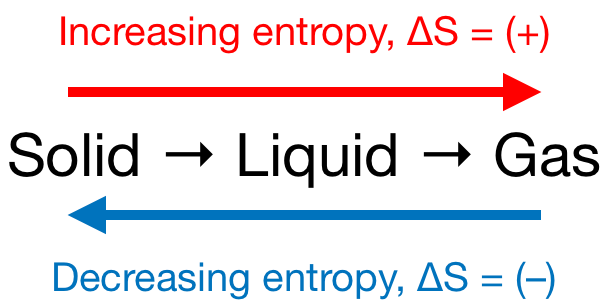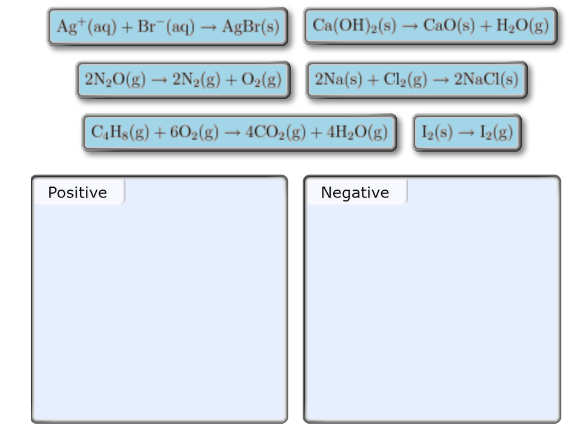Chemistry Practice Problems Entropy Practice Problems Solution: Predict the sign of the entropy change, ΔS°, for e...

🤓 Based on our data, we think this question is relevant for Professor Randles' class at UCF.

# Solution: Predict the sign of the entropy change, ΔS°, for each of the reaction displayed. Drag the appropriate items to their respective bins.

###### Problem

Predict the sign of the entropy change, ΔS°, for each of the reaction displayed. Drag the appropriate items to their respective bins.

###### Solution

We’re being asked to predict the sign of the entropy change (ΔS) for the given reactions. Recall that if:

• ΔS > 0 or ΔS = (+); entropy is increased

• ΔS < 0 or ΔS = (–); entropy is decreased

Remember that phase changes lead to a change in entropy:Also, recall that an increase in the number of gas molecules in the reaction also leads to an increase in entropy.View Complete Written SolutionEntropy

Entropy

#### Q. Predict the sign of the entropy change, ΔS°, for each of the reaction displayed.i) 2NO2 (g) → N2 (g) + 2O2 (g)ii) C5H12 (g) + 8O2 (g) → 5CO2 (g) + 6H2...

Solved • Thu Feb 22 2018 14:59:37 GMT-0500 (EST)

Entropy

#### Q. Predict the sign of the entropy change, ΔS°, for the following reaction.Pb2+ (aq) + 2Cl− (aq) → PbCl2(s)a) Negativeb) Positive                        ...

Solved • Thu Feb 22 2018 12:26:20 GMT-0500 (EST)

Entropy

#### Q. Calculate the standard entropy change for the following reaction at 25°C.2CH3OH(g) + 3O2(g) → 2CO2(g) + 4H2O(g)

Solved • Tue Feb 20 2018 11:13:43 GMT-0500 (EST)

Entropy

#### Q. The ΔH°vap for pure water is 40.65 kJ/mol. Calculate the ΔS°vap for pure water.

Solved • Mon Feb 19 2018 13:33:00 GMT-0500 (EST)